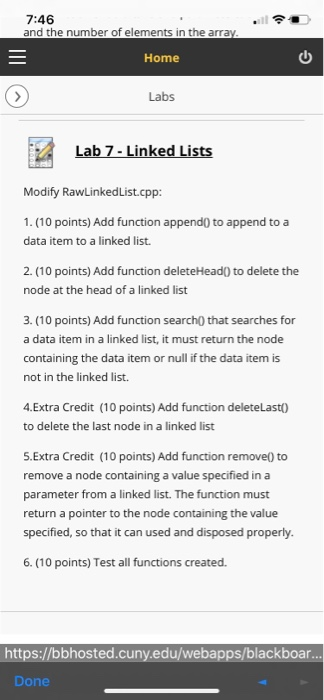# (Solved) : Demonstrates Template Function Implements Generic Version Selection Sort Algorithm Include Q42569986 . . .

//Demonstrates a template function that implements//a generic version of the selection sort algorithm.#include <iostream>using std::cout;using std::endl;
template<class T>void sort(T a[], int numberUsed);//Precondition: numberUsed <= declared size of the arraya.//The array elements a through a[numberUsed – 1] havevalues.//The assignment and < operator work for values of typeT.//Postcondition: The values of a through a[numberUsed – 1]have//been rearranged so that a <= a <=… <=a[numberUsed – 1].
template<class T>void swapValues(T& variable1, T& variable2);//Interchanges the values of variable1 and variable2.//The assignment operator must work correctly for thwe typeT.
template<class T>int indexOfSmallest(const T a[], int startIndex, intnumberUsed);//Precondition: 0 <= startIndex < numberUsed. Arrayelements have values.//The assignment and < operator work for values of typeT.//Returns the index i such that a[i] is the smallest of thevalues//a[startIndex], a[startIndex + 1],…, a[numberUsed -1].
#include “sort.cpp”
int main( ){int i;int a = {9, 8, 7, 6, 5, 1, 2, 3, 0, 4};cout << “Unsorted integers:n”;for (i = 0; i < 10; i++)cout << a[i] << ” “;cout << endl;sort(a, 10);cout << “In sorted order the integers are:n”;for (i = 0; i < 10; i++)cout << a[i] << ” “;cout << endl;double b = {5.5, 4.4, 1.1, 3.3, 2.2};cout << “Unsorted doubles:n”;for (i = 0; i < 5; i++)cout << b[i] << ” “;cout << endl;sort(b, 5);cout << “In sorted order the doubles are:n”;for (i = 0; i < 5; i++)cout << b[i] << ” “;cout << endl;
char c = {‘G’, ‘E’, ‘N’, ‘E’, ‘R’, ‘I’, ‘C’};cout << “Unsorted characters:n”;for (i = 0; i < 7; i++)cout << c[i] << ” “;cout << endl;sort(c, 7);cout << “In sorted order the characters are:n”;for (i = 0; i < 7; i++)cout << c[i] << ” “;cout << endl;
return 0;}
Modify code 16-2.cpp (which uses sort.cpp) to use a functiontemplate that displays the contents of an array.
This function should take two parameters: the array and thenumber of elements in the array.
(The function template is used to be able to handle any datatype of array).please give the solution of full code with the screenshort ofrunning..I will give thumbs up

sort.cpp
template<class T>void sort(T a[], int numberUsed){int indexOfNextSmallest;for (int index = 0; index < numberUsed – 1; index++){//Place the correct value in a[index]:indexOfNextSmallest =indexOfSmallest(a, index, numberUsed);swapValues(a[index], a[indexOfNextSmallest]);//a <= a <=…<= a[index] are the smallest ofthe original array//elements. The rest of the elements are in the remainingpositions.}}
template<class T>void swapValues(T& variable1, T& variable2){T temp;
temp = variable1;variable1 = variable2;variable2 = temp;}
template<class T>int indexOfSmallest(const T a[], int startIndex, intnumberUsed){T min = a[startIndex];int indexOfMin = startIndex;
for (int index = startIndex + 1; index < numberUsed;index++)if (a[index] < min){min = a[index];indexOfMin = index;//min is the smallest of a[startIndex] through a[index]}
return indexOfMin;}. 7:46 and the number of elements in the array. Home » Labs Labs Lab 7 – Linked Lists Modify RawLinkedList.cpp: 1. (10 points) Add function append( to append to a data item to a linked list. 2. (10 points) Add function delete Head() to delete the node at the head of a linked list 3. (10 points) Add function search() that searches for a data item in a linked list, it must return the node containing the data item or null if the data item is not in the linked list. 4.Extra Credit (10 points) Add function deleteLast() to delete the last node in a linked list 5.Extra Credit (10 points) Add function remove to remove a node containing a value specified in a parameter from a linked list. The function must return a pointer to the node containing the value specified, so that it can used and disposed properly. 6. (10 points) Test all functions created. https://bbhosted.cuny.edu/webapps/blackboar… Done Show transcribed image text . 7:46 and the number of elements in the array. Home » Labs Labs Lab 7 – Linked Lists Modify RawLinkedList.cpp: 1. (10 points) Add function append( to append to a data item to a linked list. 2. (10 points) Add function delete Head() to delete the node at the head of a linked list 3. (10 points) Add function search() that searches for a data item in a linked list, it must return the node containing the data item or null if the data item is not in the linked list. 4.Extra Credit (10 points) Add function deleteLast() to delete the last node in a linked list 5.Extra Credit (10 points) Add function remove to remove a node containing a value specified in a parameter from a linked list. The function must return a pointer to the node containing the value specified, so that it can used and disposed properly. 6. (10 points) Test all functions created. https://bbhosted.cuny.edu/webapps/blackboar… Done

Answer to //Demonstrates a template function that implements //a generic version of the selection sort algorithm.#include using st…

We are the best freelance writing portal. Looking for online writing, editing or proofreading jobs? We have plenty of writing assignments to handle.